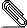# Re: [Kwant] up/down conductance

```Hi Patrik,
```
```
>  I was trying to get up/down conductance through the system
> from one end to the other, similar to the conductance from lead 0 to
>
> What would be the equivalent of conductance from lead 0 to lead 1 (in
> the 'if trans'), but for either up or down spin?

Ah, this is a new feature introduced in Kwant 1.3! The way to do this is
to first tell Kwant that your leads have a conservation law associated
with them, and to tell Kwant the basis that you want to use for the
Without this information the modes Kwant calculates in the leads will be
an arbitrary superposition of spin up and spin down, and you will not
easily be able to separate these.

You provide this information with the 'conservation_law' parameter when
Hermitian matrix with integer eigenvalues, who's eigenvectors you want
to take as the basis for the modes. The modes will be ordered by their
eigenvalues. This is explained in the docs; the example given is for
superconductivity, but the principle is the same. For your case a
reasonable choice would be sigma_z:

sym = kwant.TranslationalSymmetry([0, 0, -a])
…

There is a minus sign in front of the sigma_z so that the "up" modes
come first, and then the "down" modes.

Then, when computing the transmission, instead of saying

smatrix.transmission(1, 0)

which will give you the total transmission from lead 0 to lead 1, you
can say

smatrix.transmission((1, 0), (0, 0))

to get the transmission from the spin up block (block 0) of lead 0 to
the spin up block of lead 1. The first number in each pair is the lead
number, and the second is the block index with respect to the
conservation law you defined when creating the Builder (0 being spin up,
and 1 being spin down in this case).

Similarly you can use

smatrix.transmission((1, 1), (0, 1))

for transmission from spin down to spin down, or any other combination
to calculate the transmissions between different spin blocks.

Hope that helps,

Joe

: https://kwant-project.org/doc/1/tutorial/superconductors

```signature.asc
Description: OpenPGP digital signature